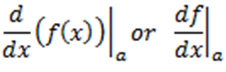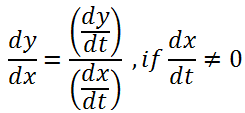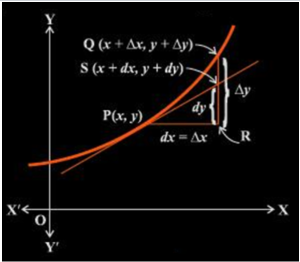# Differential Calculus and Approximation

### Differential calculus

A derivative of a function f(x) signifies the rate of change of the function f(x) with respect to x at a point “a” lying in its domain.

Also, the derivative of a function f in x, at x = a, is given as:Therefore, whenever a quantity ‘y’ varies with another quantity ‘x’ such that y = f(x), then f’(xo) indicates the rate of change of y with respect to x, at x = x0. Also, if two variables ‘x’ and ‘y’ are varying with respect to a third variable say ‘t’ then according to the chain rule, we haveTo determine the value of certain quantities differential calculus can be used very efficiently. Let a function f in x be defined such that f: D →R, D ⊂ R. Let y = f(x). Let a small increase in x be denoted by ∆x. If x increases by ∆x then the corresponding increase in y is given by ∆y = f(x + ∆x) – f(x) as shown in the figure given below.Based on the above discussion we can define the following:

• Differential of x which is represented as dx is given by dx = ∆x.
• Differential of y which is represented as dy is given by dy = f’(x)dx = (dy/dx) ∆x.

If in case the differential of x or dx = ∆x is comparatively very insignificant in comparison to x then dy is a good approximation of ∆y and dy ≈ ∆y.

From the above discussion it can be observed that differential of the independent variable is equal to the increase in the variable but on the other hand, the differential of the dependent variable is not equal to the increase of the variable.

Let us look into an example to have a better insight.

Example: Approximate $\sqrt{25.5}$ using differential.

Solution: Let us consider y = $\sqrt{x}$, where x = 25 and ∆x = 0.5. Then,

∆y = $\sqrt{x+\bigtriangleup x} -\sqrt{x}$

∆y =$\sqrt{25.5} -\sqrt{25}$

∆y = $\sqrt{25.5} – 5$

$\sqrt{25.5} = \bigtriangleup y + 5$

Since dy is approximately equal to ∆y, therefore

$dy = \frac{\mathrm{d} y}{\mathrm{d} x}\bigtriangleup x = \frac{1}{2\sqrt{x}}(0.5) = 0.05$

Therefore, the approximate value of $\sqrt{25.5} = 5 + 0.05 = 5.05$<